×
Log in to StudySoup
Get Full Access to Introductory Chemistry - 5 Edition - Chapter 6 - Problem 65p
Join StudySoup for FREE
Get Full Access to Introductory Chemistry - 5 Edition - Chapter 6 - Problem 65p

Already have an account? Login here
×
Reset your password

# Solved: For each set of molecular models, write a relationship between moles of hydrogenISBN: 9780321910295 34

## Solution for problem 65P Chapter 6

Introductory Chemistry | 5th Edition

• Textbook Solutions
• 2901 Step-by-step solutions solved by professors and subject experts
• Get 24/7 help from StudySoup virtual teaching assistantsIntroductory Chemistry | 5th Edition

4 5 1 425 Reviews
19
4
Problem 65P

Problem 65P

For each set of molecular models, write a relationship between moles of hydrogen and moles of molecules. Then determine the total number of hydrogen atoms present. (H—white; O—red; C—black; N—blue)

(a)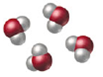(b)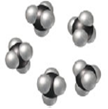(c)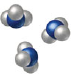Step-by-Step Solution:

Problem 65P

For each set of molecular models, write a relationship between moles of hydrogen and moles of molecules. Then determine the total number of hydrogen atoms present. (H—white; O—red; C—black; N—blue)

(a)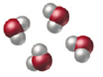(b)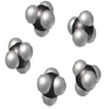(c)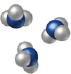Step by step solution

Step 1 of 3

a)

In the given image the compound is -One mole ofcontains two moles of “H” atoms.

Let’s calculate the number of moles of hydrogen present in the four moles of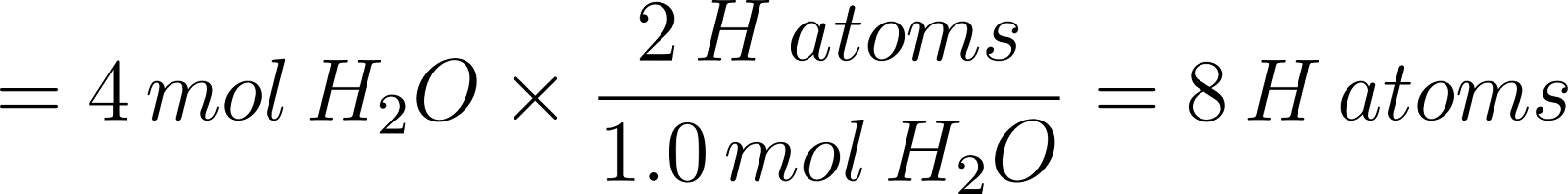Therefore, 8 H atoms present in the given sample.

___________________________________________________________________________

Step 2 of 3

Step 3 of 3

##### ISBN: 9780321910295

This textbook survival guide was created for the textbook: Introductory Chemistry, edition: 5. Introductory Chemistry was written by and is associated to the ISBN: 9780321910295. This full solution covers the following key subjects: hydrogen, Moles, Molecules, determine, atoms. This expansive textbook survival guide covers 19 chapters, and 2046 solutions. The full step-by-step solution to problem: 65P from chapter: 6 was answered by , our top Chemistry solution expert on 05/06/17, 06:45PM. Since the solution to 65P from 6 chapter was answered, more than 450 students have viewed the full step-by-step answer. The answer to “For each set of molecular models, write a relationship between moles of hydrogen and moles of molecules. Then determine the total number of hydrogen atoms present. (H—white; O—red; C—black; N—blue)(a) (b) (c)” is broken down into a number of easy to follow steps, and 32 words.

Unlock Textbook Solution

Enter your email below to unlock your verified solution to:

Solved: For each set of molecular models, write a relationship between moles of hydrogen